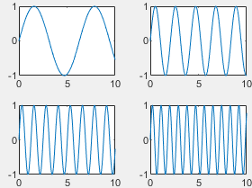# tiledlayout

## 语法

``tiledlayout(m,n)``
``tiledlayout('flow')``
``tiledlayout(___,Name,Value)``
``tiledlayout(parent,___)``
``t = tiledlayout(___)``

## 说明

``tiledlayout(m,n)` 创建分块图布局，用于显示当前图窗中的多个绘图。该布局有固定的 `m`×`n` 图块排列，最多可显示 `m*n` 个绘图。如果没有图窗，MATLAB® 会创建一个图窗并将布局放入其中。如果当前图窗包含一个现有坐标区或布局，MATLAB 会将其替换为新布局。分块图布局包含覆盖整个图窗或父容器的不可见图块网格。每个图块可以包含一个用于显示绘图的坐标区。创建布局后，调用 `nexttile` 函数以将坐标区对象放置到布局中。然后调用绘图函数在该坐标区中绘图。`

``tiledlayout('flow')` 指定布局的 `'flow'` 图块排列。最初，只有一个空图块填充整个布局。当您调用 `nexttile` 时，布局都会根据需要进行调整以适应新坐标区，同时保持所有图块的纵横比约为 4:3。`

``tiledlayout(___,Name,Value)` 使用一个或多个名称-值对组参数指定布局的其他选项。请在所有其他输入参数之后指定这些选项。例如，`tiledlayout(2,2,'TileSpacing','compact')` 创建一个 2×2 布局，图块之间采用最小间距。有关属性列表，请参阅 TiledChartLayout 属性。`

``tiledlayout(parent,___)` 在指定的父容器中而不是在当前图窗中创建布局。请在所有其他输入参数之前指定父容器。`

``t = tiledlayout(___)` 返回 `TiledChartLayout` 对象。创建布局后，使用 `t` 配置布局的属性。`

## 示例

```tiledlayout(2,2); [X,Y,Z] = peaks(20); % Tile 1 nexttile surf(X,Y,Z) % Tile 2 nexttile contour(X,Y,Z) % Tile 3 nexttile imagesc(Z) % Tile 4 nexttile plot3(X,Y,Z)``````x = linspace(0,30); y1 = sin(x/2); y2 = sin(x/3); y3 = sin(x/4); % Plot into first tile three times tiledlayout('flow') nexttile plot(x,y1)``````nexttile plot(x,y2)``````nexttile plot(x,y3)``````nexttile plot(x,y1) hold on plot(x,y2) plot(x,y3) hold off``````x = linspace(0,30); y1 = sin(x); y2 = sin(x/2); y3 = sin(x/3); y4 = sin(x/4); t = tiledlayout(2,2); % Tile 1 nexttile plot(x,y1) % Tile 2 nexttile plot(x,y2) % Tile 3 nexttile plot(x,y3) % Tile 4 nexttile plot(x,y4)``````t.TileSpacing = 'compact'; t.Padding = 'compact';``````t = tiledlayout(2,2,'TileSpacing','Compact'); % Tile 1 nexttile plot(rand(1,20)) title('Sample 1') % Tile 2 nexttile plot(rand(1,20)) title('Sample 2') % Tile 3 nexttile plot(rand(1,20)) title('Sample 3') % Tile 4 nexttile plot(rand(1,20)) title('Sample 4')``````title(t,'Size vs. Distance') xlabel(t,'Distance (mm)') ylabel(t,'Size (mm)')``````p = uipanel('Position',[.1 .2 .8 .6]); t = tiledlayout(p,2,1); % Tile 1 nexttile(t) stem(1:13) % Tile 2 nexttile(t) bar([10 22 31 43 52])``````t = tiledlayout(2,1); % First tile ax1 = nexttile; plot([1 2 3 4 5],[11 6 10 4 18]); ax1.XColor = [1 0 0]; ax1.YColor = [1 0 0]; % Second tile ax2 = nexttile; plot([1 2 3 4 5],[5 1 12 9 2],'o'); ax2.XColor = [1 0 0]; ax2.YColor = [1 0 0];````scores``strikes` 定义为包含四场保龄球联赛数据的向量。然后创建一个分块图布局，并显示三个图块，分别显示每个团队的击球数量。

```scores = [444 460 380 387 366 500 365 451 611 548 412 452]; strikes = [9 6 5 6 4 8 4 7 16 10 9 8]; t = tiledlayout('flow'); % Team 1 nexttile plot([1 2 3 4],strikes(:,1),'-o') title('Team 1 Strikes') % Team 2 nexttile plot([1 2 3 4],strikes(:,2),'-o') title('Team 2 Strikes') % Team 3 nexttile plot([1 2 3 4],strikes(:,3),'-o') title('Team 3 Strikes')``````nexttile([2 3]); bar([1 2 3 4],scores) legend('Team 1','Team 2','Team 3','Location','northwest') % Configure ticks and axis labels xticks([1 2 3 4]) xlabel('Game') ylabel('Score') % Add layout title title(t,'April Bowling League Data')````scores``strikes` 定义为包含四场保龄球联赛数据的向量。然后创建一个 `3`×`3` 分块图布局，并显示五个条形图，其中显示每个团队的击球次数。

```scores = [444 460 380 388 389 387 366 500 467 460 365 451 611 426 495 548 412 452 471 402]; strikes = [9 6 5 7 5 6 4 8 10 7 4 7 16 9 9 10 9 8 8 9]; t = tiledlayout(3,3); % Team 1 nexttile bar([1 2 3 4],strikes(:,1)) title('Team 1 Strikes') % Team 2 nexttile bar([1 2 3 4],strikes(:,2)) title('Team 2 Strikes') % Team 3 nexttile bar([1 2 3 4],strikes(:,3)) title('Team 3 Strikes') % Team 4 nexttile bar([1 2 3 4],strikes(:,4)) title('Team 4 Strikes') % Team 5 nexttile(7) bar([1 2 3 4],strikes(:,5)) title('Team 5 Strikes')``````nexttile(5,[2 2]); plot([1 2 3 4],scores,'-.') labels = {'Team 1','Team 2','Team 3','Team 4','Team 5'}; legend(labels,'Location','northwest') % Configure ticks and axis labels xticks([1 2 3 4]) xlabel('Game') ylabel('Score') % Add layout title title(t,'April Bowling League Data')``````tiledlayout(1,2) % Display geographic plot nexttile geoplot([47.62 61.20],[-122.33 -149.90],'g-*') % Display polar plot nexttile theta = pi/4:pi/4:2*pi; rho = [19 6 12 18 16 11 15 15]; polarscatter(theta,rho)````nexttile` 输出参数的一个有用的用途体现在您想调整前一个图块中的内容时。例如，您可能决定要重新配置先前绘图中使用的颜色图。

```tiledlayout(2,2); [X,Y,Z] = peaks(20); % Tile 1 nexttile surf(X,Y,Z) % Tile 2 nexttile contour(X,Y,Z) % Tile 3 nexttile imagesc(Z) % Tile 4 nexttile plot3(X,Y,Z)``````ax = nexttile(3); colormap(ax,cool)``````t = tiledlayout(2,3); [X,Y,Z] = peaks; % Tile 1 nexttile contour(X,Y,Z) % Span across two rows and columns nexttile([2 2]) contourf(X,Y,Z) % Last tile nexttile imagesc(Z)``````ax = nexttile(2); colormap(ax,hot)``````load patients tbl = table(Diastolic,Smoker,Systolic,Height,Weight,SelfAssessedHealthStatus); tiledlayout(2,2) % Scatter plot nexttile scatter(tbl.Height,tbl.Weight) % Heatmap nexttile heatmap(tbl,'Smoker','SelfAssessedHealthStatus','Title','Smoker''s Health'); % Stacked plot nexttile([1 2]) stackedplot(tbl,{'Systolic','Diastolic'});``````nexttile(1) scatterhistogram(tbl,'Height','Weight');``````Z1 = peaks; Z2 = membrane; tiledlayout(2,1); nexttile contourf(Z1) nexttile contourf(Z2)``````cb = colorbar; cb.Layout.Tile = 'east';``````t = tiledlayout('flow'); nexttile plot(rand(1,10)); nexttile plot(rand(1,10)); nexttile plot(rand(1,10));``````gax = geoaxes(t); gax.Layout.Tile = 4; gax.Layout.TileSpan = [2 3];``````geoplot(gax,[47.62 61.20],[-122.33 -149.90],'g-*') gax.MapCenter = [47.62 -122.33]; gax.ZoomLevel = 2;```## 输入参数

### 名称-值参数

`'loose'`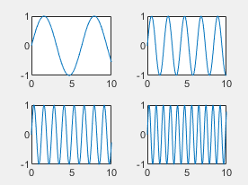`'compact'`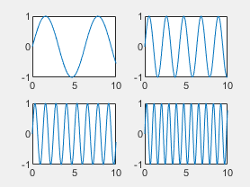`'tight'`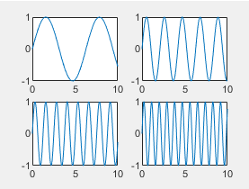`'none'`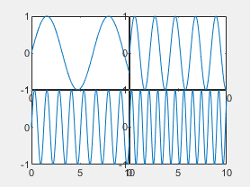`'loose'``'compact'`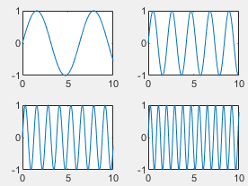`'tight'`